# How to Combine Text from Two or More Cells into One Cell

This post explains that how to combine text from two or more cells into one cell in excel. How to concatenate the text from different cells into one cell with excel formula in excel. How to join text from two or more cells into one cell using ampersand symbol. How to combine the text using the TEXTJOIN function in excel 2016.

## Combine text using Ampersand symbol

If you want to combine text from multiple cells into one cell and you can use the Ampersand (&) symbol. For example, if you want to combine texts in the Range B1:B3 into Cell C1, Just refer to the following steps:

1# Click Cell C1 that you want to put the combined text.

2# type the below formula in the formula box of C1.

`=B1&B2&B3`

3# press Enter, you will see that the text content in B1:B3 will be joined into C1.Note:

If you want to add a space between the combined text, then you can use &” ” & instead of & in the above formula.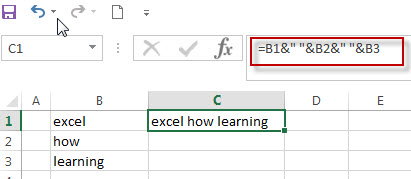And if you want to add a comma to the combined text, just type &”,”& between the combined cells in the above formula.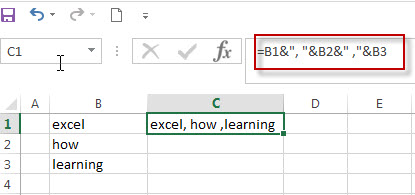If you want to add Line break between the combined cells using Ampersand operator, you should combine with the CHAR function to get the line break. Using the following formula:

`=B1 & CHAR(10) & B2 & CHAR(10) & B3`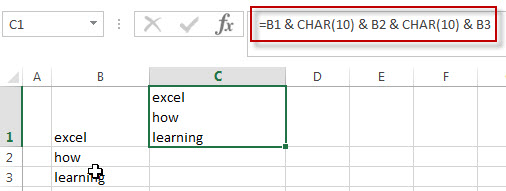## Combine text using CONCATENATE function

If you want to join the text from multiple cells into one cell, you also can use the CONCATENATE function instead of Ampersand operator. Just following the below steps to join combine the text from B1:B3 into one Cell C1.

1# Select the cell in which you want to put the combined text. (Select Cell C1)

2# type the following formula in Cell C1.

`=CONCATENATE(B1,B2,B3)`

3# press Enter to complete the formula.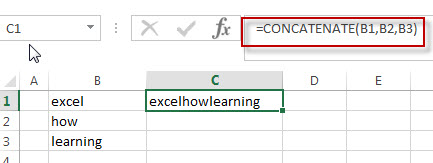Note:  If you want to add a space between the combined text strings, you can add a space character (“ “) enclosed in quotation marks. Just like the below formula:

```=CONCATENATE(B1," ",B2," ",B3)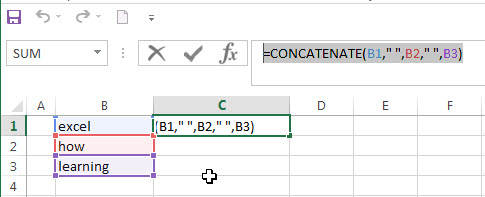```

If you want to add line break into the combined text string, you can use the CHAR function within the CONCATENATE function, just use the below formula:

`=CONCATENATE(B1,CHAR(10),B2,CHAR(10),B3)`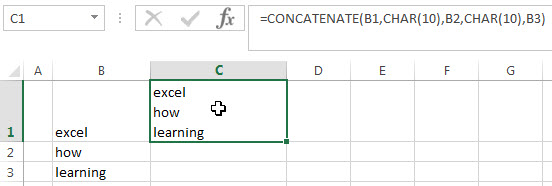## Combine text using TEXTJOIN function

If you are using the excel 2016, then you can use a new function TEXTJOIN function to combine text from multiple cells, it is only available in EXCEL 2016.  It can join the text from two or more text strings or multiple ranges into one string and also can specify a delimiter between the combined text strings. Just like the below formula:

`=TEXTJOIN(" ",TRUE,B1:B3)`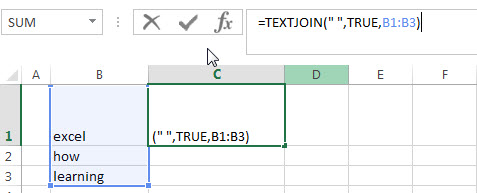### Related Formulas

• Remove Numeric Characters from a Cell
If you want to remove numeric characters from alphanumeric string, you can use the following complex array formula using a combination of the TEXTJOIN function, the MID function, the Row function, and the INDIRECT function..…
• remove non numeric characters from a cell
If you want to remove non numeric characters from a text cell in excel, you can use the array formula:{=TEXTJOIN(“”,TRUE,IFERROR(MID(B1,ROW(INDIRECT(“1:”&LEN(B1))),1)+0,””))}…

### Related Functions

• Excel Concat function
The excel CONCAT function combines 2 or more strings or ranges together.This is a new function in Excel 2016 and it replaces the CONCATENATE function.The syntax of the CONCAT function is as below:=CONCAT (text1,[text2],…)…
• Excel CHAR function
The Excel CHAR function returns the character specified by a number (ASCII Value).The CHAR function is a build-in function in Microsoft Excel and it is categorized as a Text Function. The syntax of the CHAR function is as below:=CHAR(number)….
• Excel TEXTJOIN function
The Excel TEXTJOIN function joins two or more text strings together and separated by a delimiter. you can select an entire range of cell references to be combined in excel 2016.The syntax of the TEXTJOIN function is as below:= TEXTJOIN  (delimiter, ignore_empty,text1,[text2])…